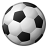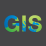# Python loop script to calculate cumulative sum based on another attribute being the same

5363
5
03-21-2016 08:44 AMNew Contributor III

I am looking for a script that will calculate the cumulative sum of say population (based on a presorted table that I have created) as long as the variable of ID is the same.  So it might look like

IDPOPCUMPOP
1100100
1250350
1150500
2100100
2500600
3150150
3600750
3100850
31501000

this:

Tags (2)
1 Solution

Accepted SolutionsbyEsri Esteemed Contributor

Hi Clinton,

Try the following:

```import arcpy
from arcpy import env
env.workspace = r"E:\Temp\Python\test.gdb"

table = "Sample"

list = []

#append IDs to list
with arcpy.da.SearchCursor(table, ["ID"]) as cursor:
for row in cursor:
list.append(row)

del cursor

#remove duplicates
list = set(list)

#update CUMPOP field
for id in list:
with arcpy.da.UpdateCursor(table, ["POP", "CUMPOP"], "ID = {0}".format(id)) as cursor:
firstTime = True
for row in cursor:
if firstTime:
row = row
cursor.updateRow(row)
newVal = row
firstTime = False
else:
print newVal
row = row + newVal
cursor.updateRow(row)
newVal = row

del cursor```
5 RepliesRegular Contributor III

Try the Dissolve—Help | ArcGIS for Desktop  tool. You could dissolve based on ID and sum on either or both of your pop fieldsbyEsri Esteemed Contributor

Hi Clinton,

Try the following:

```import arcpy
from arcpy import env
env.workspace = r"E:\Temp\Python\test.gdb"

table = "Sample"

list = []

#append IDs to list
with arcpy.da.SearchCursor(table, ["ID"]) as cursor:
for row in cursor:
list.append(row)

del cursor

#remove duplicates
list = set(list)

#update CUMPOP field
for id in list:
with arcpy.da.UpdateCursor(table, ["POP", "CUMPOP"], "ID = {0}".format(id)) as cursor:
firstTime = True
for row in cursor:
if firstTime:
row = row
cursor.updateRow(row)
newVal = row
firstTime = False
else:
print newVal
row = row + newVal
cursor.updateRow(row)
newVal = row

del cursor```New Contributor III

Thank you, this works perfectly!!New Contributor III

just for reference, I found one issue in line 33:

newVal = row

Should be:

newVal = rowNew Contributor III

Well I need the running cumulative average.  After I run this loop, I need to be able to remove or select all the rows with a value greater than or less than a certain value that I choose for my analysis.